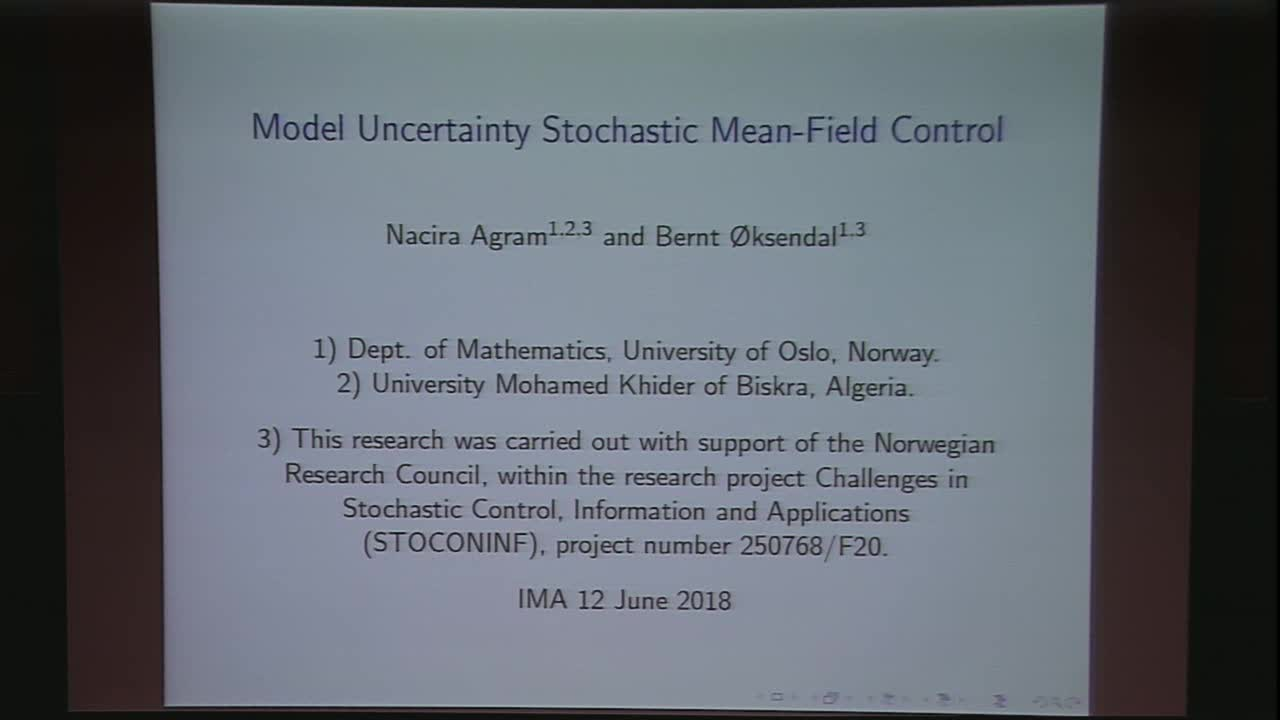## Model Uncertainty Stochastic Mean-Field Control with Applications to Finance

June 12, 2018
IMA
Presenters: Bernt Oksendal

Watch Video

#### Abstract

We consider the problem of optimal control of a mean-field stochastic differential equation (SDE) under model uncertainty. The model uncertainty is represented by ambiguity about the law L(X(t)) of the state X(t) at time t. For example, it could be the law L_P(X(t)) of X(t) with respect to the given, underlying probability measure P. This is the classical case when there is no model uncertainty. But it could also be the law L_Q(X(t)) with respect to some other probability measure Q or, more generally, any random measure \mu(t) on R with total mass 1.

We represent this model uncertainty control problem as a stochastic differential game of a mean-field related type SDE with two players. The control of one of the players, representing the uncertainty of the law of the state, is a measure-valued stochastic process \mu(t) and the control of the other player is a classical real-valued stochastic process u(t). This optimal control problem with respect to random probability processes \mu(t) in a non-Markovian setting is a new type of stochastic control problems which, to the best of our knowledge, has not been studied before.

We construct a new Hilbert space M of measures, and introduce a new type of adjoint, operator-valued BSDEs involving Fréchet derivatives with respect to measures. Using this we obtain a sufficient and a necessary maximum principle for Nash equilibria for such games in the general nonzero-sum case, and for saddle points in zero-sum games.

As an application we find an explicit solution of the problem of optimal consumption under model uncertainty of a cash flow described by a mean-field related type SDE.

The presentation is based on joint works with Nacira Agram, University of Oslo, Norway and University of Biskra, Algeria.